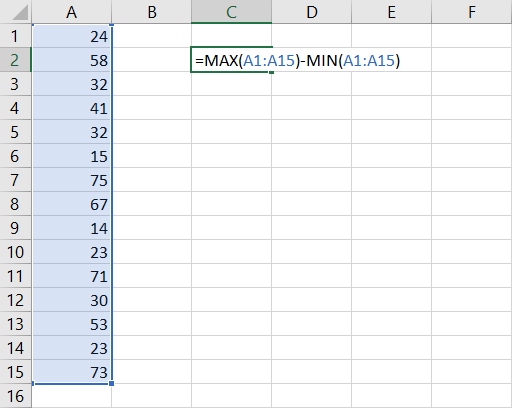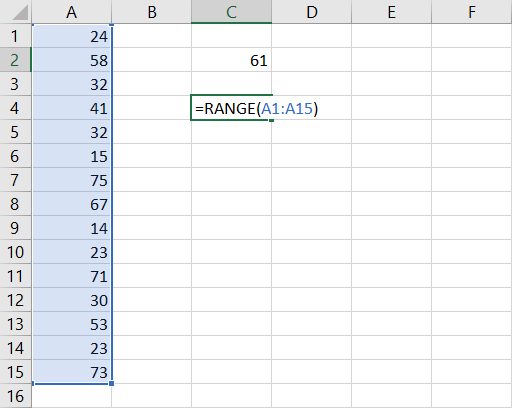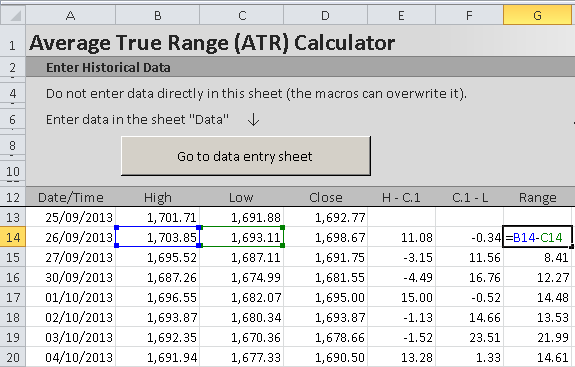# Calculating Range In Excel

Range is the difference between minimum and maximum value in a dataset. In Excel you can calculate range using the functions MIN and MAX.

For example, if you have your data in cells A1 to A15, you can calculate range in a single formula:

``=MAX(A1:15)-MIN(A1:A15)``The first part of the formula, `MAX(A1:A15)`, finds the highest value in the data (75 in the screenshot above).

The second part, `MIN(A1:15)`, finds the lowest value (14).

If you subtract the minimum from the maximum, you get range (61).

## Calculating Range in Excel VBA

Range is so commonly needed, it is surprising there is no built-in RANGE function in Excel, which would make the calculation easier than the formula above. We could just use a formula like this:

``=RANGE(A1:A15)``

Unfortunately, there is no built-in RANGE function in Excel.

However, with some basic VBA, we can create our own custom function that would work just like that.

```Function Range(Data)
' Calculates range (maximum - minimum) of data

Range = Application.WorksheetFunction.Max(Data) _
- Application.WorksheetFunction.Min(Data)

End Function```

With this function defined in VBA, we can now use it in a spreadsheet to calculate range more simply:

``=RANGE(A1:A15)``

This gives the same result as

``=MAX(A1:15)-MIN(A1:A15)``Note: Although "range" means a different, very common thing in Excel and VBA – a range of cells – it is not a reserved word in VBA and you can call your custom function RANGE. That said, you may want to consider another name to avoid any confusion, such as RNG.

## Calculating Trading Range in Excel

In the financial markets, range is the difference between highest and lowest price (high and low) in a particular time period, such as a trading day. As above, it is calculated by subtracting the minimum (low) from the maximum (high):

Range = High – Low

If you have market data in the OHLC (open-high-low-close) format in Excel, simply take the cell containing the high and subtract the cell containing the low from it – see screenshot from ATR Calculator below.Unlike most other market statistics and technical indicators, closing price does not enter the calculation of range in any way.

## Calculating Range in ATR Calculator

You can calculate average range in the ATR Calculator by selecting "Range" in the drop-down box in cells K4/L4/M4. You can also see the range for each bar in column G.

## Average True Range (ATR)

Average True Range (ATR) is a more advanced concept of range and more suitable in some types of markets. While the traditional (high minus low) range only considers the volatility (range) of the trading session itself, ATR also looks at overnight volatility (a possible price gap from the previous day's close to the current day's opening price).

Here you can see a detailed explanation of true range and ATR calculation.

By remaining on this website or using its content, you confirm that you have read and agree with the Terms of Use Agreement.

We are not liable for any damages resulting from using this website. Any information may be inaccurate or incomplete. See full Limitation of Liability.

Content may include affiliate links, which means we may earn commission if you buy on the linked website. See full Affiliate and Referral Disclosure.

We use cookies and similar technology to improve user experience and analyze traffic. See full Cookie Policy.